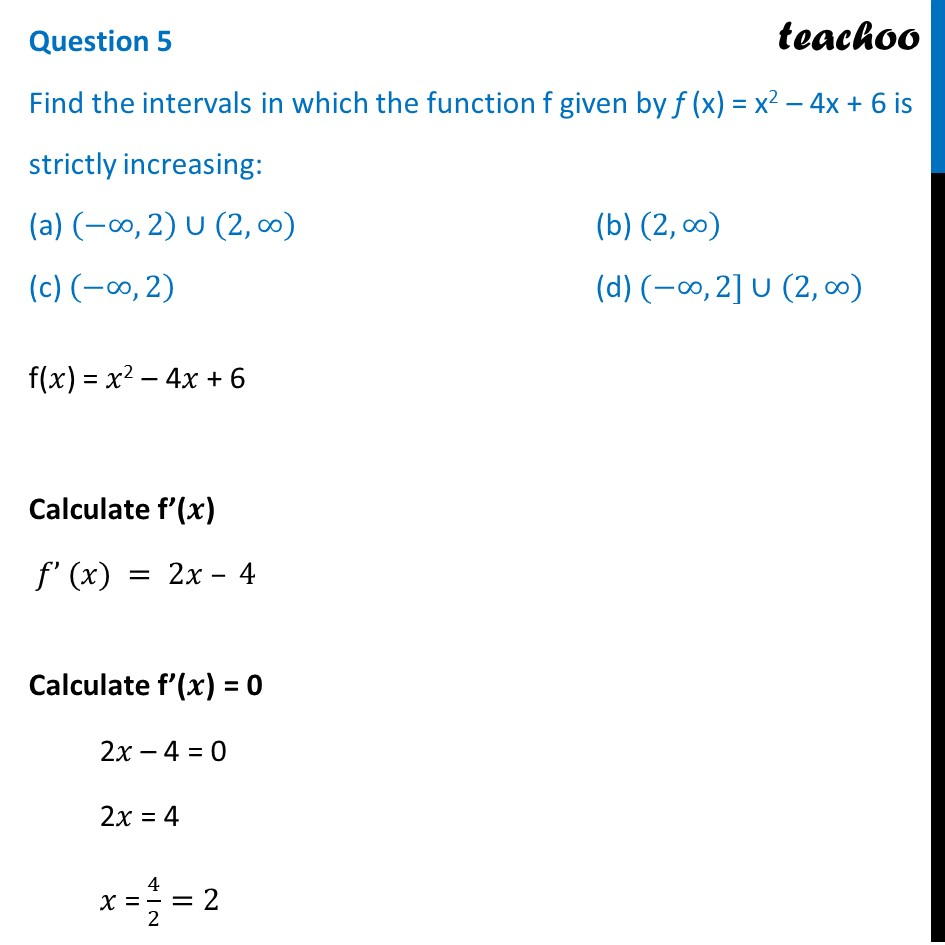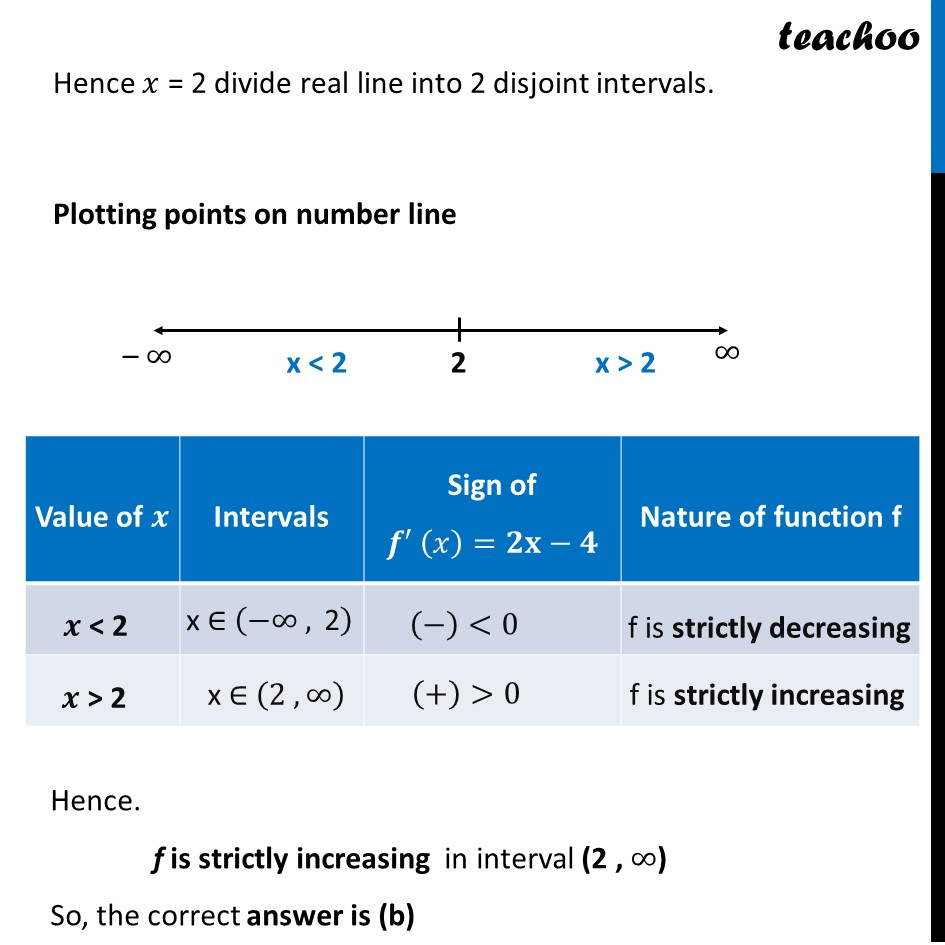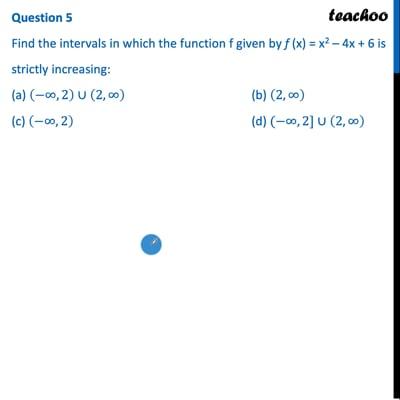CBSE Class 12 Sample Paper for 2022 Boards (MCQ Based - for Term 1)

Class 12
Solutions of Sample Papers and Past Year Papers - for Class 12 Boards

## Find the intervals in which the function f given by f (x) = x2 – 4x + 6 is strictly increasing: (a) (-∞, 2) ∪ (2,∞)   (b) (2,∞) (c) (-∞, 2)                (d) (-∞, 2] ∪ (2,∞)

This question is inspired from - Example 10 - Chapter 6 Class 12 Application of DerivativesThis video is only available for Teachoo black users

Learn in your speed, with individual attention - Teachoo Maths 1-on-1 Class

### Transcript

Question 5 Find the intervals in which the function f given by f (x) = x2 – 4x + 6 is strictly increasing: (a) (−∞, 2) ∪ (2,∞) (b) (2,∞) (c) (−∞, 2) (d) (−∞, 2] ∪ (2,∞) f(𝑥) = 𝑥2 – 4𝑥 + 6 Calculate f’(𝒙) 𝑓’ (𝑥) = 2𝑥 – 4 Calculate f’(𝒙) = 0 2𝑥 – 4 = 0 2𝑥 = 4 𝑥 = 4/2=2 Hence 𝑥 = 2 divide real line into 2 disjoint intervals. Plotting points on number line Hence. f is strictly increasing in interval (2 , ∞) So, the correct answer is (b)